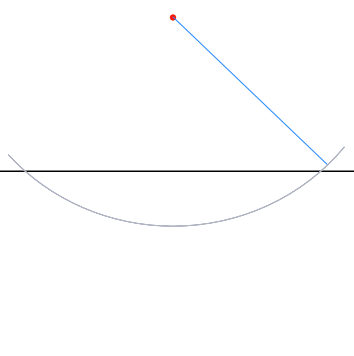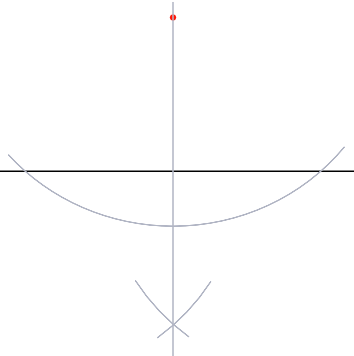Construction - Perpendicular from a point

# Construction - Perpendicular from a point

GCSE(F), GCSE(H),

To construct a perpendicular line from a given point above a base line, set the radius of the compass to be slightly more than the distance of the point to the base line.

Draw an arc with this setting to intersect the base line in two places.Without changing the radius of the compass, set the point on one of the base line intersections and draw an arc below the base line.Repeat for the other intersection.Draw a perpendicular line from that intersection through the original point.## Examples

1. A point is 16cm above a base line. The compasses are extended to a distance of 16cm between point and pencil.

Why is it not possible to draw a perpindicular from the point to the line?

Answer: The arc drawn from the point to the base line must intersect the base line in two places.

If the arc only touches the baseline, no construcion takes place and the result is likely to be less accurate.

2. How many construction lines are needed to drop a perpendicular from a point onto a base line?